## Hermitian Operator

A Hermitian Operatoris one which satisfies(1)

As shown in Sturm-Liouville Theory, ifis Self-Adjoint and satisfies the boundary conditions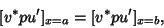(2)

then it is automatically Hermitian. Hermitian operators have Real Eigenvalues, Orthogonal Eigenfunctions, and the corresponding Eigenfunctions form a Complete set whenis second-order and linear. In order to prove that Eigenvalues must be Real and Eigenfunctions Orthogonal, consider(3)

Assume there is a second Eigenvaluesuch that(4)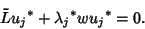(5)

Now multiply (3) byand (5) by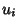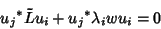(6)(7)(8)

Now integrate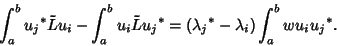(9)

But becauseis Hermitian, the left side vanishes.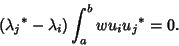(10)

If Eigenvalues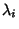andare not degenerate, then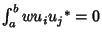, so the Eigenfunctions are Orthogonal. If the Eigenvalues are degenerate, the Eigenfunctions are not necessarily orthogonal. Now take.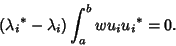(11)

The integral cannot vanish unless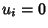, so we haveand the Eigenvalues are real.

For a Hermitian operator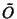,(12)

In integral notation,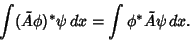(13)

Given Hermitian operators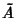and,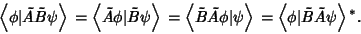(14)

Because, for a Hermitian operatorwith Eigenvalue,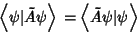(15)(16)

Therefore, eitheror. ButIff, so(17)

for a nontrivial Eigenfunction. This means that, namely that Hermitian operators produce Real expectation values. Every observable must therefore have a corresponding Hermitian operator. Furthermore,(18)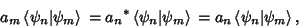(19)

since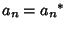. Then(20)

For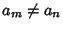(i.e.,),(21)

For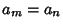(i.e.,),(22)

Therefore,(23)

so the basis of Eigenfunctions corresponding to a Hermitian operator are Orthonormal. Given two Hermitian operatorsand,(24)

the operatorequals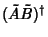, and is therefore Hermitian, only if(25)

Given an arbitrary operator,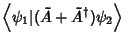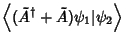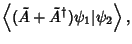(26)

sois Hermitian.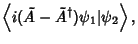(27)

sois Hermitian. Similarly,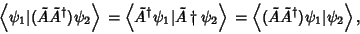(28)

so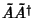is Hermitian.

Define the Hermitian conjugate operator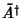by(29)

For a Hermitian operator,. Furthermore, given two Hermitian operatorsand,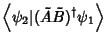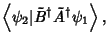(30)

so(31)

By further iterations, this can be generalized to(32)

Arfken, G. Hermitian (Self-Adjoint) Operators.'' §9.2 in Mathematical Methods for Physicists, 3rd ed. Orlando, FL: Academic Press, pp. 504-506 and 510-516, 1985.